# US6223195B1 - Discrete cosine high-speed arithmetic unit and related arithmetic unit - Google Patents

Discrete cosine high-speed arithmetic unit and related arithmetic unit Download PDF

## Info

Publication number
US6223195B1
US6223195B1 US09/460,766 US46076699A US6223195B1 US 6223195 B1 US6223195 B1 US 6223195B1 US 46076699 A US46076699 A US 46076699A US 6223195 B1 US6223195 B1 US 6223195B1
Authority
US
United States
Prior art keywords
plurality
discrete cosine
units
arithmetic unit
computer system
Prior art date
Legal status (The legal status is an assumption and is not a legal conclusion. Google has not performed a legal analysis and makes no representation as to the accuracy of the status listed.)
Expired - Fee Related
Application number
US09/460,766
Inventor
Motonobu Tonomura
Current Assignee (The listed assignees may be inaccurate. Google has not performed a legal analysis and makes no representation or warranty as to the accuracy of the list.)
Hitachi Ltd
Original Assignee
Hitachi Ltd
Priority date (The priority date is an assumption and is not a legal conclusion. Google has not performed a legal analysis and makes no representation as to the accuracy of the date listed.)
Filing date
Publication date
Priority to JP11492494A priority Critical patent/JP3652717B2/en
Priority to JP6-114924 priority
Priority to JP6-203188 priority
Priority to JP20318994A priority patent/JPH0869528A/en
Priority to JP6203188A priority patent/JPH0869450A/en
Priority to JP6-203189 priority
Application filed by Hitachi Ltd filed Critical Hitachi Ltd
Application granted granted Critical
Publication of US6223195B1 publication Critical patent/US6223195B1/en
Anticipated expiration legal-status Critical
Application status is Expired - Fee Related legal-status Critical

## Images

•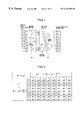•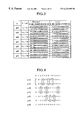•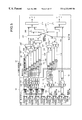•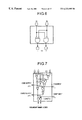•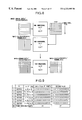•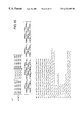•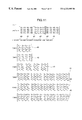•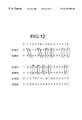•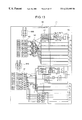•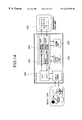•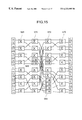•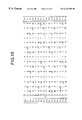•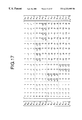•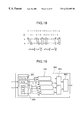•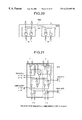•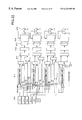•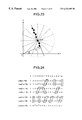## Classifications

• GPHYSICS
• G06COMPUTING; CALCULATING; COUNTING
• G06FELECTRIC DIGITAL DATA PROCESSING
• G06F17/00Digital computing or data processing equipment or methods, specially adapted for specific functions
• G06F17/10Complex mathematical operations
• G06F17/14Fourier, Walsh or analogous domain transformations, e.g. Laplace, Hilbert, Karhunen-Loeve, transforms
• G06F17/147Discrete orthonormal transforms, e.g. discrete cosine transform, discrete sine transform, and variations therefrom, e.g. modified discrete cosine transform, integer transforms approximating the discrete cosine transform

## Abstract

This arithmetic unit for carrying out partial sum of products for transform operations such as discrete cosine transform is provided which includes a plurality of first units for calculating in parallel sums of and/or differences between a plurality of input variables or sums of and/or differences between a plurality of values obtained by multiplying said plurality of input variables by a constant. The arithmetic unit also includes a processing unit having a plurality of shift units for shifting outputs from said plurality of first units by respectively predetermined numbers of digit-shifts and a plurality of second units for calculating concurrently sums of outputs from said plurality of shift units. The arithmetic can be used, for example, as a high speed discrete cosine unit, a high speed Hartley transform unit or a high speed Hough transform unit.

## Description

CROSS-REFERENCE TO RELATED APPLICATION

This application is a continuation of application Ser. No. 08/737,569, filed on Nov. 15, 1996, U.S. Pat. No. 6,029,185, the entire disclosure of which is a 371 of PCT/JP95/00953 filed May 18, 1995.

TECHNICAL FIELD

The present invention relates to an arithmetic unit of a computer system, and in particular, to a discrete cosine high-speed arithmetic unit suitable for achieving calculation of a sum of products using a plurality of constant function values and compressing and decompressing data at a high speed. Moreover, the present invention relates to a high-speed Hartley transform arithmetic unit suitable for calculating a sum of products using a plurality of constant function values and thereby executing the Hartley transform processing, which is related to a Fourier transform, at a high speed. Additionally, the present invention relates to image processing, and in particular, to a Hough transform circuit to achieve a Hough transform in which straight line components of an image are detected, the circuit being suitable for calculating a sum of products using a plurality of constant function values and executing the Hough transform processing at a high speed.

BACKGROUND ART

In voice and image processing, there has been widely employed a discrete Fourier transform (DFT) and its variations such as a discrete cosine transform and a discrete Hartley transform. In these transform processes, a plurality of trigonometric functions are utilized to primarily calculate sums of products between the trigonometric functions and data items. In general, the calculation cost of multiplication is higher than that of addition and subtraction. Consequently, there have been devised several high-speed calculation algorithms in which the number of multiplications are advantageously reduced using relationships between trigonometric functions, e.g., the formula of double angle and the formula of half-angle. These algorithms have been briefly described in pages 115 to 142 of the “Nikkei Electronics” No. 511 published on October 15, 1990. In practice, the trigonometric functions are stored as constants in a memory. Particularly, due to the relatively small number of figures of the values, there has been also adopted a method in which the results of products between data items and trigonometric functions are stored in a memory. In addition, it is possible to utilize a known method in which each trigonometric function value is calculated in a CORDIC method using the principle of rotation of coordinates and/or a formula of approximate expression of function.

In image processing, the Hough transform is often employed because the transform is advantageously applicable even when the data contains noises due to the detection of straight lines in the image. When the coordinates of an arbitrary pixel are expressed as (x,y), the Hough transform is defined as

R=x cos θ+y sin θ=x cos θ+y cos(π/2−θ).

FIG. 23 shows the geometric relationship of the transform. R stands for the length of a perpendicular drawn from the origin of the coordinate system to a straight line passing the pixel (x,y). Letter θ denotes the angle between the perpendicular and the positive direction of the x axis. In an actual application, for an arbitrary pixel, the angle θ takes a plurality of discrete values ranging from 0 to π such that R of expression (1) is calculated for each value of θ. R is also discretized and its frequency of occurrence is attained in the form of voting for all pixels so that (R, θ) having the highest number of votes obtained is detected as a straight line component.

A plurality of trigonometric functions are stored as constants in a memory for use in calculation later. Or, in the conventional method in which the value of each trigonometric function is directly calculated using, e.g., the CORDIC method, even when the number of multiplications is reduced by a clever algorithm, a considerable amount of multiplications are still necessary. Furthermore, it is not practical to provide a multiplier for each of the multiplications, namely, the multiplier is to be sequentially used. This is cause of hindrance to the high-speed operation. Additionally, since an arbitrary input is assumed in a multiplier, even when a value at a digit place of binary input data is zero, a partial product is uselessly calculated for the digit place. When there is used the method in which all of the results of products between data items and trigonometric function values are stored in the memory, although the arithmetic unit can be easily designed, the memory capacity is increased and hence the chip size becomes larger.

Moreover, to count the votes for the discrete (R, θ), there is required a large volume of memory.

DISCLOSURE OF INVENTION

It is therefore an object of the present invention to provide a discrete cosine high-speed arithmetic unit, a high-speed Hartley transform arithmetic unit, and a high-speed Hough transform circuit in which considering that each trigonometric function value is constant, to possibly minimize the number of non-zero coefficients in the binary value obtained by expanding the trigonometric function value, the value is beforehand recoded into a redundant binary representation of {−1,0,+1}. The resultant values are shifted such that a pair of non-zero coefficients is optimally grouped. For each digit position, associated data pairs are subjected to addition or subtraction according to the signs of the coefficients. Moreover, the resultant values are shifted to be aligned to a fixed position and are then inputted to a group of adders to thereby obtain partial products therebetween, thereby attaining the sum of the partial products. In consequence, the arithmetic units and circuit above are efficiently configured in a compact structure to operate at a high speed.

Since the number of non-zero coefficients is reduced in the constant and the pair of non-zero coefficient values are grouped for each digit position to commonly effect the addition in an optimal manner, the number of adders is decreased and the number of stages of gates is also minimized.

BRIEF DESCRIPTION OF DRAWINGS

FIG. 1 is a configuration diagram of a discrete cosine high-speed arithmetic unit of the present invention.

FIG. 2 is a DCT/IDCT calculation expressed in matrix form.

FIG. 3 is a table showing binary expansion values of seven cosine constants, values obtained by canonically recoding the expansion values, and values attained by multiplying the values by the square root of two.

FIG. 4 is a diagram showing a combination of variable pairs to beforehand accomplish addition or subtraction for the anterior addition and shift input positions for the posterior addition.

FIG. 5 is a circuit diagram for the calculation of the one-dimensional DCT/IDCT of the present invention.

FIG. 6 is a one-digit circuit diagram for implementing xi-xj by use of simple gates in place of adders.

FIG. 7 is a one-digit circuit diagram of a redundant binary adder employed according to the present invention.

FIG. 8 is an explanatory diagram of a method of achieving the two-dimensional DCT/IDCT by reducing the two-dimensional form into a one-dimensional form and a method of directly calculating the two-dimensional DCT/IDCT.

FIG. 9 is a table showing binary expansion values of cos α×cos β at two-dimensional points, i.e., 4×4 points and recoded values thereof.

FIG. 10 is a DCT at two-dimensional 4×4 points in matrix representation.

FIG. 11 is an IDCT at two-dimensional points 4×4 in matrix representation.

FIG. 12 is a diagram showing a method in which the DCT/IDCT at two-dimensional 4×4 points is directly calculated without reducing the two-dimensional form into a one-dimensional form and a combination of pairs of variables to beforehand accomplish addition or subtraction for the anterior addition and shift input positions for the posterior addition.

FIG. 13 is a circuit construction diagram of the present invention in which the DCT at two-dimensional 4×4 points is directly calculated without reducing the two-dimensional form into a one-dimensional form.

FIG. 14 is a configuration diagram of a chip in which a DCT/IDCT high-speed arithmetic unit of the present invention is incorporated.

FIG. 15 is a construction diagram of a high-speed Hartley transform arithmetic unit as an embodiment of the present invention.

FIG. 16 is an explanatory diagram showing a 16-point Hartley transform in matrix representation.

FIG. 17 is an explanatory diagram showing a re-arranged 16-point Hartley transform in matrix representation.

FIG. 18 is an explanatory diagram showing a state in which constants are developed into binary values.

FIG. 19 is a circuit block diagram showing a product sum circuit.

FIG. 20 is a circuit block diagram showing an initial stage of a butterfly arithmetic circuit.

FIG. 21 is a circuit block diagram showing the second and subsequent stages of the butterfly arithmetic circuit.

FIG. 22 is a construction diagram of a high-speed Hough transform circuit as an embodiment of the present invention.

FIG. 23 is an explanatory diagram showing a geometric relationship of a 16-directional Hough transform.

FIG. 24 is an explanatory diagram related to a binary expansion of constant values of cosine functions and grouping of common parts.

BEST MODE FOR CARRYING OUT THE INVENTION

A description will first be given of an 8-point discrete cosine transform (to be abbreviated as DCT herebelow). Assuming that input data and calculation data are respectively xk and Xn, the formula of DCT is expressed as follows: $X n = c n  ∑ k = 0 7   x k · cos   π   n  ( 2  k + 1 ) 16 ( 1 )$

Moreover, the formula of inverse DCT (to be abbreviated as IDCT herebelow) is expressed as: $x k = c n  ∑ n = 0 7   c n  X n · cos   π   n  ( 2  k + 1 ) 16 ( 2 )$

where, $c 0 = 1 / 2   2 ,  c n = 1 / 2   …   n ≠ 0 ( 3 )$

It is assumed g(i)=cos(πi/16), equation (1) can be represented in matrix equation as shown in FIG. 2. Additionally, equation (2) can be represented as a matrix attained by transposing the rows and columns of the matrix in FIG. 2.

Prior to conducting the DCT calculation, consider now the following formula of product sum: $X = ∑ k = 1 n   x k  a k ( 4 )$

Assuming: $a k = ∑ i = 0 m - 1   a k , i  2 i ( 5 )$

in equation (4), the following relationship results: $X = ∑ k = 1 n   x k  a k = ∑ k = 1 n   x k · (  ∑ i = 0 m - 1   a k , i  2 i ) ( 6 )$

where, ak,1ε {−1,0,30 1}. If there exist a pair of coefficients ak,i and aj,i having a relationship of ak,i=|aj,i|=1 for distinct k and j, then the following equation is obtained:

(x k a k,i +x j a j,i)2i=(x k ±x j)2i   (7)

Furthermore, if there exist a pair of coefficients ak,i and aj,m having a relationship of ak,i=|aj,m|=1 for distinct i and m, the following equation results.

(x k a k,i +x j a j,m·2m−i)2i=(x k ±x j·2m−i)2i   (8)

For the product sum xk·ak+xj·aj, if there exist a plurality of pairs of coefficients satisfying equation (7) or (8), the sums of and differences between xk and xj are calculated according to the principle represented by equation (7) and the sums of and differences between one of xk and xj and results obtained by multiplying the other one thereof by n-th power of two (n=(m−i) digit shift) are calculated according to the principle designated by equation (8), and then the obtained results are respectively shifted to digit positions to satisfy the condition above to be then added to each other, thereby reducing the number of calculations for the partial product sum.

In addition, appropriately using a relationship

2i−20=2i−1+2i−2+. . . +20   (9)

called a canonical recode in which the i non-zero coefficients can be decreased to two non-zero coefficients, there are conducted shift operations to thereby increase the pairs of coefficients satisfying equation (7) or (8).

Next, a description will be given of a method in which the amount of calculations of DCT partial product sum is minimized according to these principles. First, FIG. 3 shows a binary expansion values of seven cosine constants g(i) up to the 16-th digit. Also shown are the canonical recode values (the number of non-zero coefficients is changed from 59 to 42 through the recoding process). However, −1 is represented by applying an overline to 1. Additionally, since g(4) multiplied by the square root of two results in a simple value of one, there are also shown the results obtained by multiplying the constants by the square root of two (the number of non-zero coefficients is changed from 46 to 43). As above, applying an appropriate fixed value to the cosine constant, the number of non-zero coefficients is increased or decreased after the recoding process, and hence existence of an optimal solution can be expected. This naturally depends on the number of figures assumed. In this case, considering also the inverse DCT calculation (inversely, the constant is divided in the IDCT by the fixed value used in the multiplication) since the digit place can be easily aligned through the shift operation, it can be noted that either one of g(i) and the result obtained by multiplying g(i) by the square root of two is significant. However, this is not the case if either the DCT calculation or the IDCT calculation is utilized. In this embodiment, description will be primarily given on the assumption that the value is multiplied by the square root of two.

In the DCT calculation, g(2k+1) and g(2k) are grouped, namely, to appear respectively in an odd-numbered row and an even-numbered row as shown in FIG. 2. Furthermore, since g(k) appears as having the opposite sign in columns i and j, the difference uk=xi−xj is beforehand calculated between xi and xj. In this connection, for example, assuming u1=x0−x7, u3=x1−x6, u5=x2−x5, and u7=x3−x4 in the first row, the total sum u1·g(1)+u3·g(3)+u5·g(5)+u7·g(7)=

=1. 011000110001u1+1. 001011010000u3+0. 110010010010u6+0. 010001101010u7

(rounded up at the 13-th digit below the decimal point) is calculated as follows. First, as shown in FIG. 4, u1+u3, u1−u3, and u5+u7 are calculated in the prior addition. Thereafter, a shift and input process is carried out for the results of additions and for the digit places of appearances respectively of u1, u3, u5, and u7, thereby achieving the posterior addition for these items at once. Next, assuming u2=(x0+x7)−(x3+x4) and u6=(x1+x6)−(x2+x5) in the second row, the total sum u2·g(2)+u6·g(6)=

1. 0100111010000u2 +0. 100010101001u 6

is calculated as follows. First, as shown in FIG. 4, u2 and u6 are obtained to be added to each other in the anterior addition. Thereafter, a shift and input process is carried out for the results of additions and for the digit places of appearances respectively of u2 and u6, thereby achieving the posterior addition for these items at once. In addition, for the 0-th and 4-th rows, assuming u4=(x0+x7)+(x3+x4) and u4′=−(x1+x6)−(x2+x5), it is only necessary to first calculate u4 and u4′ to obtain the total sum (u4+u4′)·g(4)=u4+u4′. For the remaining rows, i.e., rows 3 and 6 and rows 5 and 7, the additions can be achieved by a configuration substantially similar to that used for the first and second rows. In this situation, providing selectors s1, s3, s5, s7, s2, s3, s4, and s4′ as shown in FIG. 5, the hardware of the adder section can be commonly used such that the calculation is sequentially achieved for each of the odd-numbered rows and for each of the even-numbered rows. It is to be appreciated that the hardware of the adder section is not commonly utilized, but may be disposed in a parallel fashion. That is, to concurrently calculate the total sum for each row of the matrix representation shown in FIG. 2, adder hardware is independently arranged for each row.

Next, the IDCT calculation will be described. As shown in FIG. 2, the IDCT is attained by transposing the rows and columns of the DCT in the matrix representation. The difference therebetween resides in that the g(k) appearances are grouped according to odd and even values of k in DCT, but g(k) occurs for all values of k in the DCT. However, while g(1), g(3), g(5) and g(7) appear in the 0-th column, the same items with the opposite sign occur in the seventh column. In 0-th and seventh columns, g(4), g(2), g(4), and g(6) respectively appear with the same sign. Additionally, while g(3), −g(7), −g(1), and −g(5) occur in the first column, the same items with the opposite sign appear in the sixth column. In first and sixth columns, g(4), g(6), −g(4), and −g(2) respectively appear with the same sign. While g(5), −g(1), g(7), and g(3) occur in the second-column, the same items with the opposite sign appear in the fifth column. In second and fifth columns, g(4), −g(6), −g(4), and g(2) respectively appear with the same sign. In addition, while g(7), −g(5), g(3), and −g(1) occur in the third column, the same items with the opposite sign appear in the fourth column. In the third and fourth columns, g(4), −g(2), g(4), and −g(6) respectively appear with the same sign. In consequence, for the total sum of the grouped values of g(k) related to an odd number of k, by effecting addition of the values having opposite signs for the preceding and succeeding columns, the IDCT results can be attained for two columns at the same time. Adding the circuits 240 and 241 and selectors thereof and the selectors of input data items 110 to 117 to FIG. 5, the hardware of the DCT/IDCT circuit can be commonly used.

The DCT/IDCT described above is related to one-dimensional calculations and is primarily adopted for the compression/decompression of voices. To apply the arithmetic unit to the compression/decompression of a two-dimensional image expressed with coordinates (x,y), the image is decomposed into two one-dimensional elements associated with x-directional and y-directional scans. Namely, the results of first one-dimensional elements related to the x-directional scan are provisionally stored in a random access memory (RAM). The rows and columns are transposed to be inputted to second one-dimensional elements related to the y-directional scan for the calculation. The obtained results are related to the two-dimensional image. In contrast to the conventional method described above, description will be given of a method of the present invention in which the calculation is directly achieved in the two-dimensional manner without decomposing the image in the one-dimensional elements. For simplicity of explanation, description will be given of the two-dimensional case of 4×4 points. This will be easily expanded to a case of 8×8 points. In the two-dimensional case, constant values of cos α·cos β are required to be calculated. If the fixed values respectively of cos and cos β are separately acquired for the calculation, multiplications will be required. However, in the case of 4×4 points, when the six combinations of the multiplied values are obtained in advance, the multiplications between the constants are unnecessary. Additionally, the storage operation of data in the RAM required in the conventional method in which the image is decomposed into two one-dimensional elements becomes unnecessary and hence the processing speed is increased. Assume f(i)=cos (πi/8). Then, the matrices of two-dimensional DCT and IDCT for 4×4 points are as shown in FIGS. 10 and 11. However, in this connection, the terms of coefficients which can be shifted are substantially unnecessary for the explanation and hence are not shown. Incidentally, matrix Ft indicates a transposed matrix of matrix F.

FIG. 14 shows an example of a chip system 300 adopting the DCT/IDCT high-speed arithmetic unit of the present invention. An image is stored in a buffer memory section 320 such as a frame memory or a register in which a parallel access operation can be achieved. A DCT/IDCT section 310 simultaneously obtains all data items including 8×8=64 data items necessary for the operation and then outputs results of the operation to a quantizing section 330. The data is then compressed by a variable-length encoding section 340 to be sent to a transmission route or stored on a storage media (350). Conversely, compressed data supplied from the transmission route or storage media to the system 300 is decompressed by a variable-length decoder section 340. The data is then restored into the original calculation data by an inverse quantizing section 330 to be inversely transformed by IDCT 310 into the original image data. The image data is displayed as a picture via the buffer memory 320.

Description will now be given of a 16-point discrete Hartley transform (DHT). Assuming input data and calculation data to be xk and Xn, the expression of the DHT is represented by equation (10). Moreover, the inverse equation is represented by equation (11). $X n = ∑ k = 0 15   x k · cas   2  π   nk 16   where , ( 10 ) x k = 1 16  ∑ n = 0 15   x n · cas   2  π   nk 16  ( 11 ) cas   θ = cos   θ + sin   θ ( 12 )$

Since equations (10) and (11) are of the same form when the multiplication of {fraction (1/16)} (easily implemented by a shift operation) is ignored, only equation (10) will be described in the following paragraphs. Using the equation (13) shown below, equation (10) can be represented in matrix notation as shown in FIG. 16. Moreover, the rows and columns of the matrix notation of FIG. 16 can be rearranged as shown in FIG. 17.

p=cos(π/8)+sin(π/8)=cos(π/8)+cos(3π/8), q=cos(5π/8)+sin(5π/8)=cos(3π/8)+cos(π/8)   (13)

Description will next be given of a method in which the amount of calculations of product sums is reduced in the DHT according to the principles of equations (4) to (9) described above.

In the calculation of a DHT, the values of p, q, and square root of two appear as multiplication terms in groups as shown in FIG. 17. Furthermore, since these items are of the opposite signs and occur in two or more columns il, . . . , ij, the sums of and differences between xil, . . . , xij and wk=xil± . . . ±xij are calculated beforehand in a systematic fashion.

Additionally, in relation to a row in which, for example, X1 is to be calculated, assuming

w1=x0−x8, w2=x4−x12, w3=x2−x10, w4=x1−x9, w5=x3−x11, w6=x5−x13, w7=x7−x15, and up=w4+w5, uq=w6−w7, the calculation of the total sum

w1+w2+w3·{square root over ((2+L ))}+up·p+uq·q

is classified into three calculation groups of w1+w2, w3·{square root over ((2+L ))}, and up·p+up·q. Calculation of w1+w2 is accomplished by an adder 560, w3·{square root over ((2+L ))} is calculated by a multiplier 520, and up·p+up·q is effected by a product sum unit 570.

First, in the calculation of

up·p+uq·q=up·1. 0100111001111011+uq·0. 1000101010001011

(rounded off at the 17-th digit below binary point), 2up+uq, uq−up, and uq+up are calculated in an anterior addition 555 and then a shift and input process is achieved for the results and digit places of occurrences of up and uq, thereby conducting posterior additions 552 to 554 for the resultant values at once as shown in FIGS. 18 and 19. In the calculation of W3·{square root over ((2+L ))}=w3·1.0110101000000101, w3 is shifted to be supplied to the digit position of occurrence of 1 so as to calculate the result by the group of adders (multiplier 520).

First of all, the calculating circuit of each digit place of wk=xi−xj can be configured with simple gate circuits shown in FIG. 20 without using any adder circuit because the calculations are 0−0=0, 0−1=−1, 1−0=+1, and 1−1=0.

The calculation circuit of each digit place of wk=xi+xj can be configured only in consideration of wk=xi+xi=xi−(−xj). The value of −xi can be attained by {(inverse value of xj)+1} according to the representation of two's complement. The inverse value of xj is represented by drawing an overline over xj. Furthermore, since the additions in the second and subsequent stages are the redundant binary representation of {+1,0,−1}, a basic circuit 571 shown in FIG. 21 is adopted as the redundant binary adder circuit for each digit place.

Moreover, in the Hartley transform circuit, the operation of xy, a so-called butterfly operation, is often conducted. Therefore, the gate configuration is partially shared between the arithmetic circuits of x+y and x−y to resultantly obtain a butterfly arithmetic circuit 570. The result of the total sum represented in the redundant binary representation is converted into a binary value. Since a redundant binary value can be decomposed into positive and negative binary values, the converter circuit can be easily constituted with subtracters. The subtracter may be provided with a borrow-look-back dedicated circuit which corresponds to a carry-lookahead circuit of an adder.

As a result of the description above, there is constructed a DHT arithmetic unit shown in FIG. 15. Assuming that 16 data items x0 to x15 are inputted thereto in a parallel manner, a butterfly operation is performed for the data items by eight circuits 560. Since the output therefrom is represented in redundant binary representation, the butterfly operations in the second and subsequent stages are accomplished entirely by the circuits 570. According to the groups shown in FIG. 17, the operation is subdivided into a portion including X0, X8, X4, and X12 in which the calculation is performed only by the group of butterfly arithmetic units, and a portion including X2, X10, X6, and X14 in which the calculation is carried out by a circuit 520 additionally disposed to multiply the square root of two by an input value thereto, and a portion Xi (i is an odd number) in which the calculation is executed by a circuit 550 added to the system.

Next, description will be given of a method in which the amount of calculations of partial product sum is decreased according to the principles of equations (4) to (9) already described above. Description will be given of the Hough transform in a case in which the angle θ is divided into 16 directions. Furthermore, only eight directions in the range of angle 0 to π/2 will be described. Since the minimization of the amount of calculations can be achieved by almost the same manner also for the remaining eight directions in the range of angle π/2 to π in which only the sign is partially changed, description thereof and a diagram related thereto will be omitted.

Prior to the realization of calculations of the Hough transform, consider first a formula of product sum represented by equation (14).

R=x·C x +Y·C y   (14)

Assuming now $c x = ∑ i = 0 m - 1   c x , i  2 i ,  c y = ∑ i = 0 m - 1   c y , i  2 i ( 15 )$

the following equation results: $R = x · c x + y · c y = x · ∑ i = 0 m - 1   c x , i  2 i + y · ∑ i = 0 m - 1   c y , i  2 i ( 16 )$

In the equations above, however, there is assumed a condition of cx, i, cy, iε{−1,0,+1}. If there exists a pair of coefficients of cx, i and cy, p for which cx, i=|cy,p|=1, equation (17) results:

(x·cx,i+y·cy,p·2[−i)2i=x±y·2p−i)2i   (17)

For the product sum of x·cx+y·cy, if there exist a plurality of pairs of coefficients which satisfy equation (17), the sums of and differences between x and y·2n (n=shifted by (p−i) digit places) are calculated according to the principle designated by equation (17) and then the resultant values are shifted respectively to the digit positions to satisfy the condition above so as to be added to each other, thereby decreasing the number of calculations of partial product sums.

In addition, appropriately employing the relationship of equation (18) called a canonical recoding in which i non-zero coefficients can be reduced to two non-zero coefficients, there are conducted shift operations to thereby increase the pairs of coefficients satisfying equation (17) as follows:

2i−20=2i−1+2i−2+ . . . +20   (18)

FIG. 24 shows the recoded results of eight cos values (rounded off at the 17-th digit below binary point). Moreover, according to the relationship between the equation of the Hough transform and equation (17), the common pairs of x and y for the addition and subtraction can be arranged as enclosed in a rectangle in FIG. 24.

In a case of, for example, θ=3π/16, since R x cos(3π/16)+y cos(5π/16), the calculation can be conducted in groups of x+y for the first, fourth, and 14-th digit places below binary point, x−2y for the eighth and 11-th digit places below binary point, and x+2y for the 16-th digit place below binary point in the anterior addition. In addition, these anterior addition groups can also be commonly adopted for other values of θ. In the conventional method based on multipliers, the values of cos(3π/16) and cos(5π/16) stored in a table are read therefrom to be respectively multiplied by x and y. Consequently, the common anterior addition steps above have been impossible.

Values of R to be discretized are generated by an R decoder. Moreover, the R decoder is directly connected to a voting counter such that the value of the counter associated with votes is decoded and an operation of +1 is carried out. The R decoder and voting counter are arranged for each θ.

As a result of the description above, there is configured the Hough transform circuit shown in FIG. 22. Assume that coordinate data (x,y) of an arbitrary pixel is inputted thereto. The data is subjected to additions and subtractions in the circuit 560. Since the outputs therefrom are represented in redundant binary representation, the operations in the second and subsequent stages are accomplished entirely by a circuit 571.

As an application example of the present invention, there can be considered, for example, an application in which such items primarily including straight line components as Chinese characters are to be recognized. Moreover, in a case in which when the directions are fixed to about 16 directions, there can be possibly adopted a utilization mode in which the straight line components are first detected through a coarse detection step to be then sieved for a fine detection. The sieved pixels can be further processed for a precise determination of the direction such that the operation efficiently proceeds to the subsequent work processes. For the fine determination of direction, the system conducts calculation of equation (19) according to the addition theorem of trigonometric functions, $ R = x   cos   θ + y   sin   θ = x   cos   ( α + β ) + y   cos   ( α + β ) = x  ( cos   α   cos   β + sin   αsin   β ) + y  ( sin   α   cos   β + cos   α   sin   β ) = ( x   cos   α + y   sin   α )   cos   β + ( x   sin   α + y   cos   α )   sin   β ( 19 )$

where, α is an angle with the precision of 16 divisions and β indicates an angle with a finer division. Consequently, the calculation of equation (19) is precisely achieved using multipliers in a method similar to the conventional method. Assume X=(x cos α+y sin α) and Y=(x sin α+y cos α). It can be appreciated that these values can be immediately calculated by the hardware of the present invention.

The present invention is not limited to the discrete cosine transform and Hartley transform, but can be expanded generally to trigonometric functions. Therefore, the present invention is applicable also to the discrete Fourier transform and its associated operations (such as Wavelet transform). In addition, the present invention can be applied not only to general transforms using trigonometric functions, such as Hough transforms, but also to Radon transforms which are obtained by generalizing Hough transforms. Moreover, the trigonometric functions can be expanded to general periodic functions. Additionally, the application range can be expanded to a case in which either one of the operations is a product sum operation of constants. Dimensions can be increased from two dimensions to three or more dimensions, and the discretization points can be increased to more than eight. The shift operation of the present invention is fixed as a predetermined operation. When the system to conduct the shift operation is configured with shifters, there is obtained a variable construction and hence the application range is expanded. Although a large number of adders are employed, the basic circuit of an arbitrary one digit place has a regular repetitive structure and hence the design scale can be easily increased.

INDUSTRIAL APPLICABILITY

According to the present invention, there is attained an advantage that the number of gate stages is considerably reduced and the calculation speed is increased in the DCT/IDCT, Hartley transform, and Hough transform. Moreover, since the DCT/IDCT hardware is almost all commonly used, when the basic element is repeatedly used to hold (processing speed)×(area)=(constant) for the high-speed calculation, it is possible to minimize the chip area.

## Claims (18)

What is claimed is:
1. A computer system comprising:
a discrete cosine arithmetic unit for discrete cosine transform; and
a buffer memory connected to said discrete cosine arithmetic unit,
wherein said discrete cosine arithmetic unit includes:
a plurality of first units for calculating in parallel sums of a plurality of values obtained by multiplying a plurality of input variables by a constant and differences between said plurality of values; and
a plurality of shift units for shifting outputs from said plurality of first units by respectively predetermined number of digit-shifts;
a plurality of second units for calculating sums of outputs from said plurality of shift units.
2. A computer system according to claim 1,
wherein said plurality of first units includes selecting means for selecting, for an i-th column and a j-th column of n−1=i+j in a point discrete cosine transform formula, results of calculation of sums of and differences between data of said i-th column and said j-th column immediately after said data are inputted thereto in case of a transform of said n-point discrete cosine transform formula and for selecting the inputted data of an i-th row and a j-th row itself in case of an inverse transform of n−1=1+j in said n-point discrete cosine transform formula, whereby hardware is shared between said transform and said inverse transform of said n-point discrete cosine transform formula.
3. A computer system according to claim 1, including a plurality of gate circuits, each of which is a circuit for transforming a sum in an initial data inputting stage into a subtraction to achieve 1−1=0, 1−0=1, 0−1=−1, and 0−0=0, and redundant binary adders for subsequent additions of outputs from said plurality of gate circuits.
4. A computer system according to claim 1, wherein a plurality of cosine coefficients multiplied by an appropriate fixed value of a matrix of rows and columns for discrete cosine transform are beforehand multiplied by said appropriate fixed value so that the number of non-zero coefficients after a recoding operation of said plurality of cosine coefficients of said matrix of rows and columns for discrete cosine transform is less than that of initial coefficients which would result if said plurality of cosine coefficients of said matrix of rows and columns for discrete cosine transform were not multiplied by said appropriate fixed value.
5. A computer system according to claim 1, further comprising:
a quantizing unit connected to said discrete cosine arithmetic unit; and
an encoding unit connected to said quantizing unit.
6. A computer system according to claim 5, further comprising:
a display unit connected to said buffer memory; and
a storage media connected to said encoding unit.
7. A computer system according to claim 1, further comprising:
a quantizing unit connected to said discrete cosine arithmetic unit; and
an encoding and decoding unit connected to said quantizing and inverse quantizing unit.
8. A computer system according to claim 7, further comprising:
a display unit connected to said buffer memory; and
a storage media connected to said encoding and decoding unit.
9. A computer system comprising:
a discrete cosine arithmetic unit for discrete cosine transform; and
a buffer memory connected to said discrete cosine arithmetic unit,
wherein said discrete cosine arithmetic unit includes:
a plurality of first units for pre-calculating in parallel sums of a plurality of values obtained by multiplying a plurality of input variables by a constant and/or differences between said plurality of values; and
a plurality of shift units for shifting outputs from said plurality of first units by respectively predetermined number of digit-shifts;
a plurality of second units for post-calculating sums of outputs from said plurality of shift units.
10. A computer system according to claim 9, further comprising means for beforehand calculating sums of and/or differences between a plurality of variables and values obtained by multiplying the plural variables by a constant, shifting calculated results by predetermined numbers of digit places by directly using constants resultant from the multiplications between a plurality of constants, and conducting a plurality of calculations, thereby achieving an addition for the results at a time.
11. A computer system according to claim 10, further including means for selecting, for i and j of n−1=i+j in an n-point discrete cosine transform formula, results of calculation of sums and differences between an i-th column and a j-th column immediately after data is inputted thereto in a case of transform and selecting the data itself in a case of inverse transform and means for classifying calculations thereafter into calculation of odd-numbered rows and calculation of even-numbered rows and selecting immediately before outputting results sums of and differences between the results of the groups of the even-numbered and odd-numbered rows and the results themselves, characterized in that hardware is shared between the transform and the inverse transform.
12. A computer system according to claim 10, characterized by further directly including a gate circuit for transforming an addition in an initial stage into a subtraction to achieve 1−1=0, 1−0=1, 0−1=−1, and 0−0=0 and redundant binary adders for subsequent additions.
13. A computer system according to claim 10, wherein appropriate coefficients are beforehand multiplied by a constant so that the number of non-zero coefficients after a recording operation is less than that of the initial constants.
14. A computer system according to claim 9, further comprising a section to input and to output multimedia information of voice, image, code, and the like and a memory to buffer therein data of the multimedia information for conducting parallel input and output operations of data via the buffer memory, wherein operation of the data is performed by said discrete cosine high-speed arithmetic unit.
15. A computer system according to claim 14, wherein said data operations are performed in a realtime fashion to virtually provide a storage capacity which is larger than an actual storage capacity thereof by two to three orders of magnitude.
16. A computer system according to claim 9, further comprising means for beforehand calculating sums of and/or differences between a plurality of variables and values obtained by multiplying the plural variables by a constant, shifting calculated results by a predetermined numbers of digit places by directly using a constant result determined as one constant by beforehand conducting calculation between a plurality of constants, and conducting a plurality of calculations, thereby achieving an addition for the results at a time.
17. A computer system comprising:
a discrete cosine arithmetic unit for inverse discrete cosine transform; and
a buffer memory connected to said discrete cosine arithmetic unit,
wherein said discrete cosine arithmetic unit includes:
a plurality of first units for calculating in parallel sums of a plurality of values obtained by multiplying a plurality of input variables by a constant and differences between said plurality of values; and
a plurality of shift units for shifting outputs from said plurality of first units by respectively predetermined number of digit-shifts;
a plurality of second units for calculating sums of outputs from said plurality of shift units.
18. A computer system comprising:
a discrete cosine arithmetic unit for inverse discrete cosine transform; and
a buffer memory connected to said discrete cosine arithmetic unit,
wherein said discrete cosine arithmetic unit includes:
a plurality of first units for pre-calculating in parallel sums of a plurality of values obtained by multiplying a plurality of input variables by a constant and/or differences between said plurality of values; and
a plurality of shift units for shifting outputs from said plurality of first units by respectively predetermined number of digit-shifts;
a plurality of second units for post-calculating sums of outputs from said plurality of shift units.
US09/460,766 1994-05-27 1999-12-14 Discrete cosine high-speed arithmetic unit and related arithmetic unit Expired - Fee Related US6223195B1 (en)

## Priority Applications (6)

Application Number Priority Date Filing Date Title
JP11492494A JP3652717B2 (en) 1994-05-27 1994-05-27 Discrete cosine Fast calculator
JP6-114924 1994-05-27
JP6203188A JPH0869450A (en) 1994-08-29 1994-08-29 Fast hartley transformation computing element
JP6-203189 1994-08-29
JP6-203188 1994-08-29
JP20318994A JPH0869528A (en) 1994-08-29 1994-08-29 Fast hough transforming circuit

## Related Parent Applications (2)

Application Number Title Priority Date Filing Date
US08737569 Continuation
PCT/JP1995/000953 Continuation WO1995033241A1 (en) 1994-05-27 1995-05-18 High-speed arithmetic unit for discrete cosine transform and associated operation

## Publications (1)

Publication Number Publication Date
US6223195B1 true US6223195B1 (en) 2001-04-24

# Family

## Family Applications (2)

Application Number Title Priority Date Filing Date
US08/737,569 Expired - Lifetime US6029185A (en) 1994-05-27 1995-05-18 Discrete cosine high-speed arithmetic unit and related arithmetic unit
US09/460,766 Expired - Fee Related US6223195B1 (en) 1994-05-27 1999-12-14 Discrete cosine high-speed arithmetic unit and related arithmetic unit

## Family Applications Before (1)

Application Number Title Priority Date Filing Date
US08/737,569 Expired - Lifetime US6029185A (en) 1994-05-27 1995-05-18 Discrete cosine high-speed arithmetic unit and related arithmetic unit

## Country Status (3)

US (2) US6029185A (en)
TW (1) TW284869B (en)
WO (1) WO1995033241A1 (en)

## Cited By (18)

* Cited by examiner, † Cited by third party
Publication number Priority date Publication date Assignee Title
US20010031100A1 (en) * 2000-01-24 2001-10-18 Hawley Rising Method and apparatus of reconstructing audio/video/image data from higher moment data
US6424737B1 (en) * 2000-01-24 2002-07-23 Sony Corporation Method and apparatus of compressing images using localized radon transforms
US20020111978A1 (en) * 2000-12-19 2002-08-15 Philips Electronics North America Corporation Approximate inverse discrete cosine transform for scalable computation complexity video and still image decoding
US6499045B1 (en) * 1999-10-21 2002-12-24 Xilinx, Inc. Implementation of a two-dimensional wavelet transform
US20030140079A1 (en) * 2002-01-18 2003-07-24 Murphy Charles Douglas Multiple number representations for multiplication complexity reduction in signal processing transforms
US6684235B1 (en) 2000-11-28 2004-01-27 Xilinx, Inc. One-dimensional wavelet system and method
US20040062446A1 (en) * 2002-09-26 2004-04-01 Nec Electronics Corporation Image processing apparatus supporting both discrete cosine transform and discrete wavelet transform
US20040117418A1 (en) * 2002-12-11 2004-06-17 Leonardo Vainsencher Forward discrete cosine transform engine
US20050004963A1 (en) * 1999-12-01 2005-01-06 Macronix International Co., Ltd. Parallel adder-based DCT/IDCT design using cyclic convolution
US6898583B1 (en) 2000-01-24 2005-05-24 Sony Corporation Method and apparatus of creating application-specific, non-uniform wavelet transforms
US20050114280A1 (en) * 2000-01-24 2005-05-26 Rising Hawley K.Iii Method and apparatus of using neural network to train a neural network
US20050278401A1 (en) * 2004-06-10 2005-12-15 Nec Electronics Corporation Apparatus and method for motion vector search and post filtering with reduced hardware resources
US20060244992A1 (en) * 2005-05-02 2006-11-02 Fujitsu Limited Image processor of imaging apparatus
US20070168410A1 (en) * 2006-01-11 2007-07-19 Qualcomm, Inc. Transforms with common factors
US20070200738A1 (en) * 2005-10-12 2007-08-30 Yuriy Reznik Efficient multiplication-free computation for signal and data processing
US20070233764A1 (en) * 2006-03-29 2007-10-04 Yuriy Reznik Transform design with scaled and non-scaled interfaces
US8423597B1 (en) 2003-08-29 2013-04-16 Nvidia Corporation Method and system for adaptive matrix trimming in an inverse discrete cosine transform (IDCT) operation
US9798698B2 (en) 2012-08-13 2017-10-24 Nvidia Corporation System and method for multi-color dilu preconditioner

## Families Citing this family (10)

* Cited by examiner, † Cited by third party
Publication number Priority date Publication date Assignee Title
WO2000022551A1 (en) * 1998-10-13 2000-04-20 Chris Cheah Method and system for controlled distribution of information over a network
JP2001346213A (en) * 2000-06-02 2001-12-14 Nec Corp Discrete cosine transform unit and its discrete cosine transform method
EP1368748A2 (en) * 2001-01-10 2003-12-10 Philips Electronics N.V. Method and system to encode a set of input values into a set of coefficients using a given algorithm
US7634159B2 (en) * 2004-12-08 2009-12-15 Motorola, Inc. System and method for parallel computation of an array transform
US9110849B2 (en) 2009-04-15 2015-08-18 Qualcomm Incorporated Computing even-sized discrete cosine transforms
US9069713B2 (en) 2009-06-05 2015-06-30 Qualcomm Incorporated 4X4 transform for media coding
US9118898B2 (en) 2009-06-24 2015-08-25 Qualcomm Incorporated 8-point transform for media data coding
US9081733B2 (en) 2009-06-24 2015-07-14 Qualcomm Incorporated 16-point transform for media data coding
US9075757B2 (en) 2009-06-24 2015-07-07 Qualcomm Incorporated 16-point transform for media data coding
US9824066B2 (en) 2011-01-10 2017-11-21 Qualcomm Incorporated 32-point transform for media data coding

## Citations (11)

* Cited by examiner, † Cited by third party
Publication number Priority date Publication date Assignee Title
JPS60218168A (en) 1984-04-13 1985-10-31 Fujitsu Ltd Space filter circuit
US4791598A (en) 1987-03-24 1988-12-13 Bell Communications Research, Inc. Two-dimensional discrete cosine transform processor
JPH02125366A (en) 1988-07-15 1990-05-14 Honda Motor Co Ltd Arithmetic unit for rotary motion recurring formula
JPH0335353A (en) 1989-07-01 1991-02-15 Toshiba Corp Discrete cosine converting device
JPH0520457A (en) 1991-07-12 1993-01-29 A T R Shichiyoukaku Kiko Kenkyusho:Kk Multiplex resolution processing incorporating type hough transformation method
US5249146A (en) 1991-03-27 1993-09-28 Mitsubishi Denki Kabushiki Kaisha Dct/idct processor and data processing method
JPH05268481A (en) 1992-03-18 1993-10-15 Casio Comput Co Ltd Picture compressing expansion equipment
US5357453A (en) 1992-12-30 1994-10-18 Electronics And Telecommunications Research Institute Discrete cosine transform circuit
US5477469A (en) 1992-11-12 1995-12-19 Nec Corporation Operation device and operation method for discrete cosine transform and inverse discrete cosine transform
US5598361A (en) 1993-10-26 1997-01-28 Kabushiki Kaisha Toshiba Discrete cosine transform processor
US5737256A (en) 1994-10-13 1998-04-07 Fujitsu Limited Inverse discrete cosine transform apparatus

## Patent Citations (11)

* Cited by examiner, † Cited by third party
Publication number Priority date Publication date Assignee Title
JPS60218168A (en) 1984-04-13 1985-10-31 Fujitsu Ltd Space filter circuit
US4791598A (en) 1987-03-24 1988-12-13 Bell Communications Research, Inc. Two-dimensional discrete cosine transform processor
JPH02125366A (en) 1988-07-15 1990-05-14 Honda Motor Co Ltd Arithmetic unit for rotary motion recurring formula
JPH0335353A (en) 1989-07-01 1991-02-15 Toshiba Corp Discrete cosine converting device
US5249146A (en) 1991-03-27 1993-09-28 Mitsubishi Denki Kabushiki Kaisha Dct/idct processor and data processing method
JPH0520457A (en) 1991-07-12 1993-01-29 A T R Shichiyoukaku Kiko Kenkyusho:Kk Multiplex resolution processing incorporating type hough transformation method
JPH05268481A (en) 1992-03-18 1993-10-15 Casio Comput Co Ltd Picture compressing expansion equipment
US5477469A (en) 1992-11-12 1995-12-19 Nec Corporation Operation device and operation method for discrete cosine transform and inverse discrete cosine transform
US5357453A (en) 1992-12-30 1994-10-18 Electronics And Telecommunications Research Institute Discrete cosine transform circuit
US5598361A (en) 1993-10-26 1997-01-28 Kabushiki Kaisha Toshiba Discrete cosine transform processor
US5737256A (en) 1994-10-13 1998-04-07 Fujitsu Limited Inverse discrete cosine transform apparatus

## Non-Patent Citations (5)

* Cited by examiner, † Cited by third party
Title
IEICE Transactions on Fundamentals of Electronics, Communications and Computer Sciences, vol. E78-A, No. 8, Aug. 1995, pp. 957-962.
Japanese Journal, "Nikkei Electronics", No. 511, Oct. 15, 1990, pp. 115-142.
Potkonjak et al., "Efficient Substitution of Multiple Constant Multiplications by Shifts and Additions Using Iterative Pairwise Matching", 31st Design Automation Conference, San Diego, CA, Proceedings 1994, Jun. 6-10, 1994, pp. 189-194.
Technical Report of The Institute of Electronics, Information and Communication Engineers, Sep. 1994, pp. 39-46.
Transactions of Information Processing Society of Japan, vol. 31, No. 8 (Tokyo), Oct. 15, 1993, pp. 1242-1250.

## Cited By (31)

* Cited by examiner, † Cited by third party
Publication number Priority date Publication date Assignee Title
US6499045B1 (en) * 1999-10-21 2002-12-24 Xilinx, Inc. Implementation of a two-dimensional wavelet transform
US20070094320A1 (en) * 1999-12-01 2007-04-26 Macronix International Co., Ltd. Parallel Adder-Based DCT / IDCT Design Using Cyclic Convolution
US6871208B1 (en) * 1999-12-01 2005-03-22 Macronix International Co., Ltd. Parallel adder-based DCT/IDCT design using cyclic convolution
US20050004963A1 (en) * 1999-12-01 2005-01-06 Macronix International Co., Ltd. Parallel adder-based DCT/IDCT design using cyclic convolution
US7117236B2 (en) 1999-12-01 2006-10-03 Macronix International Co. Ltd Parallel adder-based DCT/IDCT design using cyclic convolution
US6876779B2 (en) 2000-01-24 2005-04-05 Sony Côrporation Method and apparatus of reconstructing audio/video/image data from higher moment data
US6898583B1 (en) 2000-01-24 2005-05-24 Sony Corporation Method and apparatus of creating application-specific, non-uniform wavelet transforms
US7213008B2 (en) 2000-01-24 2007-05-01 Sony Corporation Method and apparatus of creating application-specific, non-uniform wavelet transforms
US6976012B1 (en) 2000-01-24 2005-12-13 Sony Corporation Method and apparatus of using a neural network to train a neural network
US20010031100A1 (en) * 2000-01-24 2001-10-18 Hawley Rising Method and apparatus of reconstructing audio/video/image data from higher moment data
US6424737B1 (en) * 2000-01-24 2002-07-23 Sony Corporation Method and apparatus of compressing images using localized radon transforms
US20050114280A1 (en) * 2000-01-24 2005-05-26 Rising Hawley K.Iii Method and apparatus of using neural network to train a neural network
US6684235B1 (en) 2000-11-28 2004-01-27 Xilinx, Inc. One-dimensional wavelet system and method
US20020111978A1 (en) * 2000-12-19 2002-08-15 Philips Electronics North America Corporation Approximate inverse discrete cosine transform for scalable computation complexity video and still image decoding
US6859815B2 (en) * 2000-12-19 2005-02-22 Koninklijke Philips Electronics N.V. Approximate inverse discrete cosine transform for scalable computation complexity video and still image decoding
US20030140079A1 (en) * 2002-01-18 2003-07-24 Murphy Charles Douglas Multiple number representations for multiplication complexity reduction in signal processing transforms
US7346640B2 (en) * 2002-09-26 2008-03-18 Nec Electronics Corporation Image processing apparatus supporting both discrete cosine transform and discrete wavelet transform
US20040062446A1 (en) * 2002-09-26 2004-04-01 Nec Electronics Corporation Image processing apparatus supporting both discrete cosine transform and discrete wavelet transform
US20040117418A1 (en) * 2002-12-11 2004-06-17 Leonardo Vainsencher Forward discrete cosine transform engine
US7792891B2 (en) * 2002-12-11 2010-09-07 Nvidia Corporation Forward discrete cosine transform engine
US8423597B1 (en) 2003-08-29 2013-04-16 Nvidia Corporation Method and system for adaptive matrix trimming in an inverse discrete cosine transform (IDCT) operation
US20050278401A1 (en) * 2004-06-10 2005-12-15 Nec Electronics Corporation Apparatus and method for motion vector search and post filtering with reduced hardware resources
US7813015B2 (en) * 2005-05-02 2010-10-12 Fujitsu Semiconductor Limited Image processor of imaging apparatus
US20060244992A1 (en) * 2005-05-02 2006-11-02 Fujitsu Limited Image processor of imaging apparatus
US20070200738A1 (en) * 2005-10-12 2007-08-30 Yuriy Reznik Efficient multiplication-free computation for signal and data processing
US20070168410A1 (en) * 2006-01-11 2007-07-19 Qualcomm, Inc. Transforms with common factors
US8595281B2 (en) 2006-01-11 2013-11-26 Qualcomm Incorporated Transforms with common factors
US20070233764A1 (en) * 2006-03-29 2007-10-04 Yuriy Reznik Transform design with scaled and non-scaled interfaces
US8849884B2 (en) 2006-03-29 2014-09-30 Qualcom Incorporate Transform design with scaled and non-scaled interfaces
US9727530B2 (en) 2006-03-29 2017-08-08 Qualcomm Incorporated Transform design with scaled and non-scaled interfaces
US9798698B2 (en) 2012-08-13 2017-10-24 Nvidia Corporation System and method for multi-color dilu preconditioner

## Also Published As

Publication number Publication date
WO1995033241A1 (en) 1995-12-07
TW284869B (en) 1996-09-01
US6029185A (en) 2000-02-22

## Similar Documents

Publication Publication Date Title
Jiang et al. The spatial relationship of DCT coefficients between a block and its sub-blocks
Yu et al. DCT implementation with distributed arithmetic
US4791598A (en) Two-dimensional discrete cosine transform processor
US5590067A (en) Method and arrangement for transformation of signals from a frequency to a time domain
DE69838390T2 (en) Improved device and method for modular multiplication and exponentiation based on montgomerymultiplikation
Sorensen et al. On computing the discrete Hartley transform
He et al. A new approach to pipeline FFT processor
US5053985A (en) Recycling dct/idct integrated circuit apparatus using a single multiplier/accumulator and a single random access memory
US7218789B2 (en) Method for lossless encoding of image data by approximating linear transforms and preserving selected properties for image processing
US5301136A (en) Method and apparatus for fast implementation of inverse discrete cosine transform in a digital image processing system using low cost accumulators
Swarztrauber FFT algorithms for vector computers
EP0902375B1 (en) Apparatus for fast Fourier transform
EP0736205B1 (en) Method and apparatus for performing a fast hadamard transform
US4777614A (en) Digital data processor for matrix-vector multiplication
US5224062A (en) Method and apparatus for fast implementation of inverse discrete cosine transform in a digital image processing system using optimized lookup tables
US4489393A (en) Monolithic discrete-time digital convolution circuit
US6073154A (en) Computing multidimensional DFTs in FPGA
US4760543A (en) Orthogonal transform processor
Arai et al. A fast DCT-SQ scheme for images
EP0083967A2 (en) Monolithic fast Fourier transform circuit
JP2866754B2 (en) Processor
EP0412252B1 (en) System and circuit for the calculation of a bidimensional discrete transform
Zeng et al. Integer DCTs and fast algorithms
US5467131A (en) Method and apparatus for fast digital signal decoding
US20030115233A1 (en) Performance optimized approach for efficient downsampling operations

## Legal Events

Date Code Title Description
REMI Maintenance fee reminder mailed
LAPS Lapse for failure to pay maintenance fees
FP Expired due to failure to pay maintenance fee

Effective date: 20050424